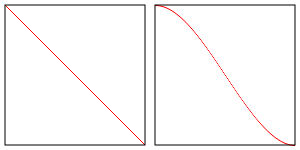# WebGLFundamentals.org

Fix, Fork, Contribute

# WebGL 三维聚光灯

``````            限制值
角度   |   弧度   | 点乘空间
--------+---------+----------
0    |   0.0   |    1.0
22   |    .38  |     .93
45   |    .79  |     .71
67   |   1.17  |     .39
90   |   1.57  |    0.0
180   |   3.14  |   -1.0
``````

``````dotFromDirection = dot(surfaceToLight, -lightDirection)
if (dotFromDirection >= limitInDotSpace) {
// 使用光照
}
``````

``````precision mediump float;

// 从顶点着色器传入的值
varying vec3 v_normal;
varying vec3 v_surfaceToLight;
varying vec3 v_surfaceToView;

uniform vec4 u_color;
uniform float u_shininess;
+uniform vec3 u_lightDirection;
+uniform float u_limit;          // 在点乘空间中

void main() {
// 因为 v_normal 是可变量，被插值过
// 所以不是单位向量，单位可以让它成为再次成为单位向量
vec3 normal = normalize(v_normal);

vec3 surfaceToLightDirection = normalize(v_surfaceToLight);
vec3 surfaceToViewDirection = normalize(v_surfaceToView);
vec3 halfVector = normalize(surfaceToLightDirection + surfaceToViewDirection);

-  float light = dot(normal, surfaceToLightDirection);
+  float light = 0.0;
float specular = 0.0;

+  float dotFromDirection = dot(surfaceToLightDirection,
+                               -u_lightDirection);
+  if (dotFromDirection >= u_limit) {
*    light = dot(normal, surfaceToLightDirection);
*    if (light > 0.0) {
*      specular = pow(dot(normal, halfVector), u_shininess);
*    }
+  }

gl_FragColor = u_color;

// 只将颜色部分（不包含 alpha） 和光照相乘
gl_FragColor.rgb *= light;

// 直接加上高光
gl_FragColor.rgb += specular;
}
``````

``````  var lightDirection = [?, ?, ?];

...

var lightDirectionLocation = gl.getUniformLocation(program, "u_lightDirection");
var limitLocation = gl.getUniformLocation(program, "u_limit");
``````

``````    gl.uniform3fv(lightDirectionLocation, lightDirection);
gl.uniform1f(limitLocation, Math.cos(limit));
``````

``````function step(a, b) {
if (b >= a) {
return 1;
} else {
return 0;
}
}
``````

``````  float dotFromDirection = dot(surfaceToLightDirection,
-u_lightDirection);
// 如果光线在聚光灯范围内 inLight 就为 1，否则为 0
float inLight = step(u_limit, dotFromDirection);
float light = inLight * dot(normal, surfaceToLightDirection);
float specular = inLight * pow(dot(normal, halfVector), u_shininess);
``````

``````-uniform float u_limit;          // 在点乘空间中
+uniform float u_innerLimit;     // 在点乘空间中
+uniform float u_outerLimit;     // 在点乘空间中

...

float dotFromDirection = dot(surfaceToLightDirection,
-u_lightDirection);
-  float inLight = step(u_limit, dotFromDirection);
+  float limitRange = u_innerLimit - u_outerLimit;
+  float inLight = clamp((dotFromDirection - u_outerLimit) / limitRange, 0.0, 1.0);
float light = inLight * dot(normal, surfaceToLightDirection);
float specular = inLight * pow(dot(normal, halfVector), u_shininess);
``````

GLSL 也有一个函数可以稍微做一些简化，叫做 `smoothstep`，和 `step` 相似返回一个 0 到 1 之间的值，但是它获取最大和最小边界值，返回该值在边界范围映射到 0 到 1 之间的插值。

`````` smoothstep(lowerBound, upperBound, value)
``````

``````  float dotFromDirection = dot(surfaceToLightDirection,
-u_lightDirection);
-  float limitRange = u_innerLimit - u_outerLimit;
-  float inLight = clamp((dotFromDirection - u_outerLimit) / limitRange, 0.0, 1.0);
float inLight = smoothstep(u_outerLimit, u_innerLimit, dotFromDirection);
float light = inLight * dot(normal, surfaceToLightDirection);
float specular = inLight * pow(dot(normal, halfVector), u_shininess);
``````### 小心 GLSL 中的 undefined 情况

GLSL 中的一些函数在某些情况下会出现 undefined，对一个负值使用`pow`求幂就是一个例子， 因为结果是无法预期。我们遇到的另一个例子就是上方的`smoothstep`

``genType asin (genType x)``

``genType acos (genType x)``

``genType atan (genType y, genType x)``

``genType pow (genType x, genType y)``

``genType log (genType x)``

``genType log2 (genType x)``

``genType sqrt (genType x)``

``genType inversesqrt (genType x)``

``````genType clamp (genType x, genType minVal, genType maxVal)
genType clamp (genType x, float minVal, float maxVal)``````

``````genType smoothstep (genType edge0, genType edge1, genType x)
genType smoothstep (float edge0, float edge1, genType x)``````

``` genType t;
t = clamp ((x – edge0) / (edge1 – edge0), 0, 1);
return t * t * (3 – 2 * t);
```

• 基础概念
• 图像处理
• 二维平移，旋转，缩放和矩阵运算
• 三维
• 光照
• 组织和重构
• 几何
• 纹理
• 渲染到纹理
• 阴影
• 技术
• 建议
• 优化
• 杂项
• 参考

Issue/Bug? 在GitHub上提issue.